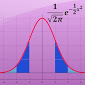# Mathematics: Recommended Resources

## Math Web Sources

Math Help

Videos

Open Resource Alternatives to MatLab

## Search Box Central

• How numbers work: discover the strange and beautiful  world of mathematics
• 50 Maths Ideas You Really Need to Know
• Basic mathematics: an introduction
• Teach yourself: basic mathematics: an introduction
• The Princeton Companion to Mathematics

The BC3 Library has several options for finding articles on chemistry topics in addition to the SciFinder database.

You will be able to find some full text articles in Academic Search Premier, PubMed, and Google Scholar, but many of the articles in all of these databases will need to be requested using the BC3 Library's Article Request form. This is a free service for BC3 students and employees.Google Scholar includes both books and articles. Be careful when selecting that the source is reputable. If an article is not made freely available online, remember that you can use the BC3 Library's Request an Article service to request it and we will do our best to obtain it and email it to you - free of charge.

## Featured Books## Books

The BC3 Library has both print and electronic books for mathematics. All of the print books and many of the ebooks are listed in the BC3 Library Catalog where you will find Dewey call numbers to locate print books on the shelves (lower level of the library) and links to connect you to e-books. E-books can also be searched separately in our two e-book collections: e-Book Central by Proquest and e-Book Collection by EBSCO.

Browsing the Stacks

You will find books related to engineering primarily in the 500 section of the library

510s -  Mathematics. Here is a sketch of some of the different relevant classification numbers for the books.

• 510 - Mathematics philosophy and theory
• 512 - Agebra
• 516 - Geometry
• 519 - Probability and applied mathematics
• Game theory
• Statistics
• Programming

## Math AppsMathematics-Includes formula calculation with variables, sums, products and sequences; solve equations (linear, quadratic, cubic, transpose); plot functions, derivate, integrate, calculate roots and extremas (polynomial, rational, exponential, etc); and more. (Android)FX Calculus Problem Solver-Guide you on how to solve your Calculus homework and textbook problems. (iOS)Khan Academy: PreCalculus-Allows students to learn PreCalculus through various videos which are download directly to their devices. (iOS)Hands-On Equations-Helps students to learn the basics of algebra. (Android)Statistics Calculator-Functions include: statistics, statistical distributions, and frequency tables. (Android)

## ConnectRequest an ARTICLERequest a BOOK or VIDEOAsk a questionSchedule an appointment